International
Tables for
Crystallography
Volume C
Mathematical, physical and chemical tables
Edited by E. Prince

International Tables for Crystallography (2006). Vol. C, ch. 6.1, pp. 592-593

## Section 6.1.2.5. The scattering cross section for polarized neutrons

P. J. Browna

#### 6.1.2.5. The scattering cross section for polarized neutrons

| top | pdf |

The cross section for scattering of neutrons with an arbitrary spin direction is obtained from (6.1.2.9)but adding also nuclear scattering given by the nuclear structure factor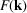, which is assumed to be spin independent. In this case,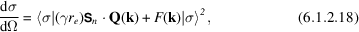the scattering without change of spin direction is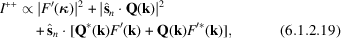and, for the spin flip scattering,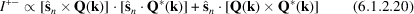with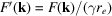.

The cross section I++ implies interference between the nuclear and the magnetic scattering when both occur for the same k. This interference is exploited for the production of polarized neutrons, and for the determination of magnetic structure factors using polarized neutrons.

In the classical method for determining magnetic structure factors with polarized neutrons (Nathans, Shull, Shirane & Andresen, 1959), the flipping ratio' R, which is the ratio between the cross sections for oppositely polarized neutrons, is measured: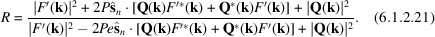In this equation,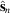is a unit vector parallel to the polarization direction. P is the neutron polarization defined as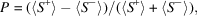where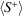and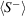are the expectation values of the neutron spin parallel and antiparallel toaveraged over all the neutrons in the beam. e is the flipping efficiency' defined as e = (2f − 1), where f is the fraction of the neutron spins that are reversed by the flipping process. Equation (6.1.2.21)is considerably simplified when bothand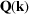are real and the polarization direction is parallel to the magnetization direction, as in a sample magnetized by an external field. The `flipping ratio' then becomes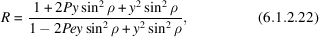with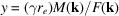, ρ being the angle between the magnetization direction and the scattering vector. The solution to this equation is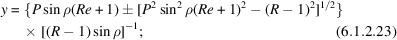the relative signs ofand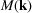are determined by whether R is greater or less than unity. The uncertainty in the sign of the square root in (6.1.2.23)corresponds to not knowing whether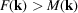or vice versa.

### References

Nathans, R., Shull, C. G., Shirane, G. & Andresen, A. (1959). The use of polarised neutrons in determining the magnetic scattering by iron and nickel. J. Phys. Chem. Solids, 10, 138–146.Google Scholar